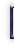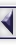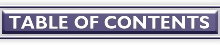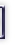# Numerical Workbenches, part II

#### By Christoph Spiel

In Part I , we looked at the most basic operations of the numerical workbenches GNU/Octave 2.1.34, Scilab 2.6, and Tela 1.32. This time we will talk about matrices, have a look at some of the predefined functions, learn how to write our own functions, and introduce flow control statements. The article closes with a brief discussion of the applications' input and output facilities.

## Matrices

Vectors help a lot if data depend on a single parameter. The different parameter values are reflected by different index values. If data depend on two parameters, vectors are a clumsy container and a more general structure, which allows for two independent indices is needed. Such a structure is called a matrix. Matrices are packed like a fresh six-pack: they are rectangular storage containers and no bottle -- oops -- element is missing.

Matrices are, for example, built from scalars as the next transcript of a GNU/Octave session demonstrates.

```    octave:1> #               temperature    rain    sunshine
octave:1> #                  degF       inches     hours
octave:1> weather_data = [    73.4,       0.0,     10.8;  ...
>                             70.7,       0.0,      8.5;  ...
>                             65.2,       1.3,      0.7;  ...
>                             68.2,       0.2,      4.1]
weather_data =
```
```      73.40000   0.00000  10.80000
70.70000   0.00000   8.50000
65.20000   1.30000   0.70000
68.20000   0.20000   4.10000
```

Three new ideas appear in the example. First, we have introduced some comments to label the columns of our matrix. A comment starts with a pound sign ```#`'' and extends until the end of the line. Second, the rows of a matrix are separated by semi-colons ```;`'', and third, if an expression stretches across two or more lines, the unfinished lines must end with the line-continuation operator ```...`''.

Similarly to vectors, matrices can not only be constructed from scalars, but from vectors or other matrices. If we had some variables holding the weather data of each day, like

```    weather_mon = [73.4, 0.0, 10.8]
weather_tue = [70.7, 0.0,  8.5]
weather_wed = [65.2, 1.3,  0.7]
weather_thu = [68.2, 0.2,  4.1]
```

we would have defined `weather_data` with

```    weather_data = [weather_mon; weather_tue; weather_wed; weather_thu]
```

or, on the other hand, if we had the data from the various instruments as

```    temperature = [73.4; 70.7; 65.2; 68.2]
rain = [0.0; 0.0; 1.3; 0.2]
sunshine = [10.8; 8.5; 0.7; 4.1]
```

we would have defined `weather_data` with

```    weather_data = [temperature, rain, sunshine]
```

The fundamental rule is: Commas separate columns, semi-colons separate rows.

The scalars living in matrix `m` are accessed by applying two indices: `m(row, column)`, where row is the row-index, and column is the column index. Thus, the amount of rain fallen on Wednesday is fetched with the expression

```    octave:10> weather_data(3, 2)
ans = 1.3000
```

Entries are changed by assigning to them:

```    octave:11> weather_data(3, 2) = 1.1
weather_data =
```
```      73.40000   0.00000  10.80000
70.70000   0.00000   8.50000
65.20000   1.10000   0.70000
68.20000   0.20000   4.10000
```

Now that we have defined `weather_data` we want to work with it. We can apply all binary operations that we have seen in last month's article on vectors. However, for this particular example, computing

```    rain_forest_weather_data = weather_data + 2.1
siberian_summer_weather_data = weather_data / 3.8
```

does not make much sense, though the computer will not complain at all. In the first example it would dutifully add `2.1` to every element of `weather_data`, in the second it would -- obedient like a sheepdog -- divide each element by `3.8`.

Say we want to do something meaningful to `weather_data` and convert all temperatures from degrees Fahrenheit to degrees Celsius. To that end, we need to access all elements in the first column. The vector of interest is

```    octave:16>     temp = [weather_data(1, 1); ...
>                      weather_data(2, 1); ...
>                      weather_data(3, 1); ...
>                      weather_data(4, 1)]
temp =
```
```      73.400
70.700
65.200
68.200
```

Obviously, the row-indices `[1, 2, 3, 4]` form a vector themselves. We can use a shortcut and write this vector of indices as

```    temp = weather_data([1, 2, 3, 4], 1)
```

In general, any vector may be used as index vector. Just watch out that no index is out of range. Ordering of the indices does matter (for example ``` weather_data([2, 1, 4, 3], 1)``` puts Tuesday's temperature in front) and repeated indices are permitted (for example ```weather_data([3, 3, 3, 3, 3, 3, 3], 1)``` holds Wednesday's temperature seven times).

In our example, the index-vector can be generated by a special built-in, the range generation operator ```:`''. To make a vector that starts at low and contains all integers from low to high, we say

```    low:high
```

For example

```    octave:1> -5:2
ans =
```
```      -5  -4  -3  -2  -1   0   1   2
```

Our weather data example now simplifies to

```    temp = weather_data(1:4, 1)
```

Accessing a complete column or row is so common that further shortcuts exist. If we drop both, low and high from the colon-operator, it will generate all valid column indices for us. Therefore, we reach at the shortest form to get all elements in the first column.

```    octave:17> temp = weather_data(:, 1)
temp =
```
```      73.400
70.700
65.200
68.200
```

With our new knowledge, we extract the sunshine hours on Tuesday, Wednesday, and Thursday

```    octave:19> sunnyhours = weather_data(2:4, 3)
sunnyhours =
```
```      8.50000
0.70000
4.10000
```

and Tuesday's weather record

```    octave:20> tue_all = weather_data(2, :)
tue_all =
```
```      70.70000   0.00000   8.50000
```

Now it is trivial to convert the data on the rain from inches to millimeters: Multiply the second column of `weather_data` by 25.4 (Millimeters per Inch) to get the amount of rain in metric units:

```    octave:21> rain_in_mm = 25.4 * weather_data(:, 2)
rain_in_mm =
```
```       0.00000
0.00000
27.94000
5.08000
```

We have already seen that vectors are compatible with scalars

```    1.25 + [0.5, 0.75, 1.0]
```

or

```    [-4.49, -4.32, 1.76] * 2
```

Scalars are also compatible with matrices.

```    octave:1> 1.25 + [ 0.5,   0.75, 1.0; ...
>                 -0.75,  0.5,  1.25; ...
>                 -1.0,  -1.25, 0.5]
ans =
```
```      1.75000  2.00000  2.25000
0.50000  1.75000  2.50000
0.25000  0.00000  1.75000
```
```    octave:2> [-4.49, -4.32, 1.76; ...
>           9.17,  6.35, 3.27] * 2
ans =
```
```       -8.9800   -8.6400    3.5200
18.3400   12.7000    6.5400
```

In each case the result is the scalar applied to every element in the vector or matrix.

How about vectors and matrices? Obviously, an expressions like

```    [7, 4, 9] + [3, 2, 7, 6, 6]
[2, 4; 1, 6] - [1, 1, 9, 4]
```

do not make any sense. In the first line the vectors disagree in size (3 vs. 5 elements), in the second line they have different shapes (2 columns and 2 rows vs. 4 columns and 1 row). To make sense, vectors or matrices that are used in an addition or subtraction must have the same shape, which means the same number of rows and the same number of columns. The technical term for ``shape'' in this context is dimension. We can query the dimension of anything with the built-in function ``` size()```.

```    octave:22> size(weather_data)
ans =
```
```      4  3
```
```    octave:23> size(sunnyhours)
ans =
```
```      3  1
```

The answer is a vector whose first element is the number of rows, and whose second element is the number of columns of the argument.

Multiplication and division of matrices can be defined in two flavors, both of which are implemented in the numerical workbenches.

• Element by element multiplication or division of two vectors or matrices of same dimensions: The number in the first row and first column of the first matrix is multiplied by the number in the first row and first column of the second matrix and so on for every element.
```    a = [3, 3; ...
6, 4; ...
6, 3]
b = [9, 3; ...
8, 2; ...
0, 3]
```
```    octave:1> a .* b
ans =
```
```      27   9
48   8
0   9
```

The element-by-element operators are preceded by a dot: element-by-element multiplication ```.*`'' and element-by-element division ```./`''.

• Matrix multiplication as known from Linear Algebra: c = a * b, where a is a p-times-q matrix and b is a q-times-r matrix. The result c is a p-times-r matrix.

Example:

```    a = [3, 3; ...
6, 4; ...
6, 3]
```
```    b = [-4,  0, 1, -4; ...
-1, -3, 2,  0]
```
```    octave:1> a * b
ans =
```
```      -15   -9    9  -12
-28  -12   14  -24
-27   -9   12  -24
```

Although we have not seen `for`-loops yet (they will be discussed farther down), I would like to write the code behind the matrix multiplication operator ```*`'' to give the reader an impression of the operations involved.

```    for i = 1:p
for j = 1:r
sum = 0
for k = 1:q
sum = sum + a(i, k)*b(k, j)
end
c(i, j) = sum
end
end
```

Compare these triply nested `for`-loops with the simple expression `c = a * b`.

• Matrix division? You cannot divide by a matrix! However, operator ```/`'' is defined for vectors and matrices. But writing x = b / a, where a and b are matrices or vectors has nothing to do with division at all! It means: please solve the system of linear equations
```    x * a = b
```

for x, given matrix a and the right-hand-side(s) b. Here ```*`'' denotes matrix multiplication as defined in the previous item, and the same rules for compatible dimensions of a and b apply.

```    a = [-2, 3,  1; ...
7, 8,  6; ...
2, 0, -1]
```
```    b = [-26,  5, -6; ...
24, 53, 26]
```
```    octave:1> x = b / a
x =
```
```       7.00000  -2.00000   1.00000
7.00000   4.00000   5.00000
```

Isn't that an easy way to solve a system of linear equations? Imagine you had to write the code which does exactly that.

Finally, let us verify the result by multiplying with a again

```    octave:2> x*a
ans =
```
```      -26.0000    5.0000   -6.0000
24.0000   53.0000   26.0000
```

which, as expected, recovers b.

Details

• For convenience GNU/Octave and Scilab define an alternative matrix division operator ```\`''. x =  a \ b solves the linear system of equations
```    a * x = b
```

for x, given matrix a and the right-hand-side(s) b. This is the form most users prefer, because here x is a column vector, whereas operator ```/`'' returns x as row-vector.

• operator ```\`'' has the dotted cousin ```.\`'' and the relation a ./ b ==  b .\ a holds.

Differences

• Scilab and Tela use C++-like comments
```    // This is a Scilab or a Tela comment
```
• Tela does not need or understand the line continuation operator ```...`''
```    weather_data = #(73.4, 0.0, 10.8;
70.7, 0.0,  8.5;
65.2, 1.3,  0.7;
68.2, 0.2,  4.1)
```

In interactive mode, Tela does not handle multi-line expressions as the above. Multi-line expressions must be read from a file (with ``` source("filename.t")```).

• In Tela the operators ```*`'' and ```/`'' work element by element, this is, they work like ```.*`'' and ```./`'' do in GNU/Octave and Scilab. Matrix multiplication (a * b in GNU/Octave or Scilab) is written as
```    a ** b
```

or

```    matmul(a, b)
```

solving systems of linear equations (b / a in Octave or Scilab) as

```    linsolve(a, b)
```

## Built-In Matrix Functions

Ugh -- far too many to mention! The workbenches supply dozens of predefined functions. Here I can only wet the reader's appetite.

Generating Special Matrices
Several matrices occur often enough in computations that they have been given their own generating functions. These are for example, m-times-n matrices filled with zeros: `zeros(m, n)` or ones: `ones(m, n)`, or n-times-n diagonal matrices, where the diagonal consists entirely of ones: `eye(n)` or the diagonal is set to numbers supplied in a vector: ```diag([a1, a2, ..., I<an>])```.
Analyzing Matrices
Getting the smallest or largest element in matrix a: ``` min(a)```, `max(a)`, or totaling matrix a: ``` sum(a)```.

Differences: GNU/Octave's `min(a)`, ``` max(a)```, and `sum(a)` return the column-wise result as a row vector. To get the minimum, maximum, and sum of all elements in matrix a, use `min(min(a))`, ``` max(max(a))```, `sum(sum(a))`.

Linear Algebra
We mentioned that systems of linear equations, like x * a = b, are solved for x with the slash operator ```/`''. But many more linear algebra functions exist, for example singular value decomposition: ``` svd(a)```, or eigenvalue computation: `eig(a)`.

Differences: In Tela uses `SVD(a)` instead of `svd(a)`, and instead of `eig(a)`, Scilab uses ``` spec(a)``` to compute the eigenvalue spectrum.

One note on performance: basically, all three applications are interpreters. This means that each expression is first parsed, then the interpreter performs desired computations, finally calling the functions inside of the expressions -- all in all a relatively slow process in comparison to a compiled program. However, functions like those shown above are used in their compiled form! They execute almost at top speed. What the interpreter does in these cases is to hand over the complete matrix to a compiled Fortran, C, or C++ function, let it do all the work, and then pick up the result.

Thus we deduce one of the fundamental rules for successful work with numerical workbenches: prefer compiled functions over interpreted code. It makes a tremendous difference in execution speed.

## User Defined Functions

No matter how many functions a program may provide its users, they are never enough. Users always need specialized functions to deal with their problems, or they simply want to group repeated, yet predefined operations. In other words, there always is a need for user-defined functions.

User functions are best defined in files, so that they can be used again in later sessions. For GNU/Octave, functions files end in .m, and are loaded either automagically or with `source("filename.m")`. Scilab calls its function files .sci, and requires them to be loaded with ``` getf("filename.sci")```. Tela functions are stored in .t-files and loaded with ``` source("filename.t")```. As big as the differences are in loading functions, all workbenches use quite similar syntax for the definition of functions.

GNU/Octave and Scilab

```    function [res1, res2, ..., resM] = foo(arg1, arg2, ..., argN)
# function body
endfunction
```

Tela

```    function [res1, res2, ..., resM] = foo(arg1, arg2, ..., argN)
{
// function body
};
```

where arg1 to argN are the functions' arguments (also known as parameters), and res1 to resN are the return values. Yes, trust your eyes, multiple return values are permitted, what might come as a surprise to most readers who are acquainted with popular programming languages. However, this is a necessity, as no function is allowed to change any of its input arguments.

Enough theory! let us write a function that takes a matrix as input and returns a matrix of the same dimensions, with the entries rescaled to lie in the interval (0, 1).

```    ### Octave
```
```    function y = normalize(x)
## Return matrix X rescaled to the interval (0, 1).
```
```        minval = min(min(x))
maxval = max(max(x))
```
```        y = (x - minval) / (maxval - minval)
endfunction
```

Now define a Scilab function that returns the spectral radius on a matrix. We use `abs()` which returns the magnitude of its (possibly complex) argument.

```    // Scilab
```
```    function r = spectral_radius(m)
// Return the spectral radius R of matrix M.
```
```        r = max(abs(spec(m)))
endfunction
```

Finally, we write a Tela function which computes the Frobenius norm of a matrix.

```    // Tela
```
```    function x = frobenius(m)
// Return the Frobenius norm X of matrix M.
{
x = sqrt(sum(abs(m)^2))
};
```

Details:

GNU/Octave's ``automagical'' function file loading works the following way: if Octave runs into an undefined function name it searches the list of directories specified by the built-in variable `LOADPATH` for files ending in .m that have the same base name as the undefined function; for example, `x = my_square_root(2.0)` looks for the file my_square_root.m in the directories listed in `LOADPATH`.

## Flow Control Statements

All code we have written thus far executes strictly top-to-bottom, we have not used any flow control statements such as conditionals or loops.

Before we manipulate the flow of control, we should look at logical expressions because the conditions used in conditionals and loops depend on them. Logical expressions are formed from (1.) numbers, (2.) comparisons, and (3.) logical expressions catenated with logical operators.

1. Zero means logically false, any number not equal to zero means logically true, hence C-programmers should feel at home.
2. The usual gang of comparison operators exist: less-than ```<`'', less-or-equal ```<=`'', greater-than ```>`'', greater-or-equal ```>=`'', and equal ```==`''.

Differences: The inequality operator varies quite a bit among the programs. (Octave cannot decide whether it feels like C, Smalltalk, or Pascal. Scilab wants to be Smalltalk and Pascal at the same time. :-)

```    !=   ~=   <>    # Octave
~=   <>         // Scilab
!=              // Tela
```
3. Complex logical expressions are formed with logical operators ``and'', ``or'' and ``not'' whose syntax is borrowed from C. However, each program uses its own set of operators. Thus, we have to list some

Differences:

```    and      or     not
----    ----    ----
&       |      !  ~     # Octave
&       |      ~        // Scilab
&&      ||     !        // Tela
```

We are all set now for the first conditional, the ``` if```-statement. Note that the parenthesis around the conditions are mandatory (as they are in C). The `else`-branches are optional in any case.

```    # Octave                // Scilab               // Tela
```
```    if (cond)               if cond then            if (cond) {
# then-body             // then-body            // then-body
else                    else                    } else {
# else-body             // else-body            // else-body
endif                   end                     };
```

cond is a logical expression as described above.

`while`-statements:

```    # Octave                // Scilab               // Tela
```
```    while (cond)            while cond              while (cond) {
# body                  // body                 // body
endwhile                end                     };
```

Again, cond is a logical expression.

`for`-statements in Octave and Scilab walk through the columns of expr one by one. Most often expr will be a vector generated with the range operator ```:`'', like ```for i = 1:10```. Tela's `for`-statement is the same as C's.

```    # Octave                // Scilab               // Tela
```
```    for var = expr          for var = expr          for (init; cond; step) {
# body              // body                     // body
endfor                  end                     };
```

Here come some examples which only show things we have discussed so far.

Octave

```    function n = catch22(x0)
## The famous catch-22 function: it is
## impossible to compute that it will
## stop for a specific input.  Returns
## the number of loops.
```
```        n = 0
x = x0
while (x != 1)
if (x - floor(x/2)*2 == 0)
x = x / 2
else
x = 3*x + 1
endif
n = n + 1
endwhile
endfunction
```

Scilab

```    function m = vandermonde(v)
// Return the Vandermonde matrix M based on
// vector V.
```
```        [rows, cols] = size(v)
m = []                      // empty matrix
if rows < cols then
for i = 0 : (cols-1)
m = [m; v^i]
end
else
for i = 0 : (rows-1)
m = [m, v^i]
end
end
endfunction
```

Tela

```    function vp = sieve(n)
// Sieve of Erathostenes; returns vector of
// all primes VP that are strictly less than
// 2*N.  1 is not considered to be a prime
// number in sieve().
{
vp = #();               // empty vector
if (n <= 2) { return };
```
```        vp = #(2);
flags = ones(1, n + 1);
for (i = 0; i <= n - 2; i = i + 1)
{
if (flags[i + 1])
{
p = i + i + 3;
vp = #(vp, p);
for (j = p + i; j <= n; j = j + p)
{
flags[j + 1] = 0
}
}
}
};
```

## Input/Output

We have been using with the workbenches a lot. At some point we would like to call it a day, but we do not want to lose all of our work. Our functions are already stored in files. It is time to see how to make our data persist.

### Simple Input and Output

All three applications at least have one input/output (I/O) model that borrows heavily from the C programming language. This model allows close control of the items read or written. Often though, it is unnecessary to take direct control over the file format written. If variables must be saved just to be restored later, simplified I/O commands will do.

• Octave offers the most flexible solution with the ``` save```/`load` command pair.
```    save filename varname1 varname2 ... varnameN
```

saves the variables named varname1, varname2, ..., varnameN in file filename. The complementary

```    load filename varname1 varname2 ... varnameN
```

command restores them from filename. If `load` is given no variable names, all variables form filename are loaded. Handing over names to `load` selects only the named variables for loading.

Note that the `save` and `load` commands do not have parenthesis and their arguments are separated by spaces not commas. Filename and variable names are strings.

```    save "model.oct-data" "prantl" "reynolds" "grashoff"
```

By default `load` does not overwrite existing variables, but complain with an error if the user tries to do so. When it is save to discard of the values of existing variables, add option ```-force`'' to `load`, like

```    load -force "model.oct-data" "reynolds"
```

and variable `reynolds` will be loaded from file model.oct-data no matter whether it has existed before or not.

• Scilab's simple I/O parallels that of GNU/Octave:
```    save(filename, var1, var2, ..., varN)
```

However, the variables var1, ..., varN are not strings, but appear literally. This means that the name of a variable is not stored in the file. The association between name and contents is lost!

The complementary function

```    load(filename, varname1, varname2, ..., varnameN)
```

restores the contents of filename in the variables named varname1, varname2, ... varnameN.

• Tela lets the users save her variables with the
```    save(filename, varname1, varname2, ..., varnameN)
```

function, preserving the association between variable name and variable contents. The complementary

```    load(filename)
```

function loads all variables stored in filename. It is not possible to select specific variables.

### Matrix Oriented I/O

As we use matrices so often, specialized functions exist to load and save whole matrices. Especially loading a matrix with a single command is convenient and efficient to read data from experiments or other programs.

Let us assume, we have the ASCII file datafile.ascii which contains the lines

```    # run 271
# 2000-4-27
#
# P/bar   T/K     R/Ohm
# ======  ======  ======
19.6      0.118352  0.893906e4
15.9846   0.1  0.253311e5
39.66     0.378377  0.678877e4
13.6      0.752707  0.00622945e4
12.4877   0.126462  0.61755e5
```

and sits in the current working directory. The file's five leading lines are non-numeric. They are skipped by the workbenches, but possibly aid the user in identifying her data. I have intentionally taken a data set which is not neatly formatted, as are most data files. Matrix-loading functions split the input at whitespace not at a specific column, thus they are happy with datafile.ascii.

We load the data into GNU/Octave with

```    octave:1> data = load("datafile.ascii")
data =
```
```       1.9600e+01   1.1835e-01   8.9391e+03
1.5985e+01   1.0000e-01   2.5331e+04
3.9660e+01   3.7838e-01   6.7888e+03
1.3600e+01   7.5271e-01   6.2294e+01
1.2488e+01   1.2646e-01   6.1755e+04
```

or into Scilab

```    -->data = fscanfMat("datafile.ascii")
data  =
```
```    !   19.6       0.118352    8939.06 !
!   15.9846    0.1         25331.1 !
!   39.66      0.378377    6788.77 !
!   13.6       0.752707    62.2945 !
!   12.4877    0.126462    61755.  !
```

or into Tela

```    >data1 = import1("datafile.ascii")
>data1
#(      19.6,  0.118352,   8939.06;
15.9846,       0.1,   25331.1;
39.66,  0.378377,   6788.77;
13.6,  0.752707,   62.2945;
12.4877,  0.126462,     61755)
```

In all three examples data will contain a 5-times-3 matrix with all the values from datafile.ascii.

The complementary commands for saving a single matrix in ASCII format are

```    save("data.ascii", "data")                # GNU/Octave
fprintfMat("data.ascii", data, "%12.6g")  // Scilab
export_ASCII("data.ascii", data)          // Tela
```

Note that Scilab's `fprintfMat()` requires a third parameter that defines the output format with a C-style template string.

Of course none of the above save commands writes the original header, the lines starting with hash-symbols, of datafile.ascii. To write these, we need the ``low-level'', C-like input/output functions, which featured in each of the three workbenches.

### C-like Input/Output

For a precise control of the input and the output, C-like I/O models are offered. All three applications implement function

```    printf(format, ...)
```

Moreover, GNU/Octave and Tela follow the C naming scheme with their C-style file I/O:

```    handle = fopen(filename)
fprintf(handle, format, ...)
fclose(handle)
```

whereas Scilab prefixes these functions with an ```m`'' instead of an ```f`''

```    handle = mopen(filename)
mprintf(handle, format, ...)
mclose(handle)
```

Whether the function is called `fprintf()` or ``` mprintf()```, they work the same way.

Next Month: Graphics, function plotting and data plotting.

####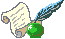Christoph Spiel

Chris runs an Open Source Software consulting company in Upper Bavaria/Germany. Despite being trained as a physicist -- he holds a PhD in physics from Munich University of Technology -- his main interests revolve around numerics, heterogenous programming environments, and software engineering. He can be reached at cspiel@hammersmith-consulting.com.

##### Copyright © 2001, Christoph Spiel. Copying license http://www.linuxgazette.net/copying.html Published in Issue 70 of Linux Gazette, September 2001